# Back to unit 9 triangles! Triangles are named by their sides or by their angles

 Date conversion 27.04.2018 Size 30.46 Kb.

### Vertex

1) The vertex of an angle is the point where the two rays that form the angle intersect.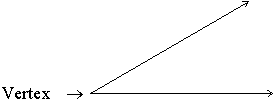2) The vertices of a polygon are the points where its sides intersect.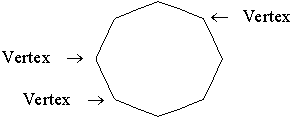### Triangle

A three-sided polygon. The sum of the angles of a triangle is 180 degrees.

Examples:### Equilateral Triangleor Equiangular Triangle

A triangle having all three sides of equal length. The angles of an equilateral triangle all measure 60 degrees.

Examples: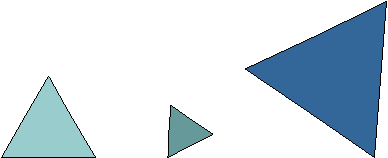### Isosceles Triangle

A triangle having two sides of equal length.

Examples: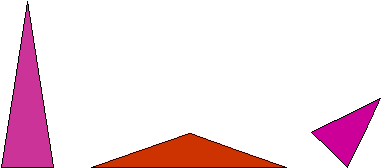### Scalene Triangle

A triangle having three sides of different lengths.

Examples:### Acute Triangle

A triangle having three acute angles.

Examples: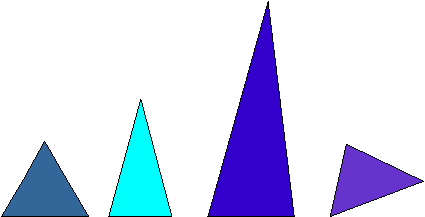### Obtuse Triangle

A triangle having an obtuse angle. One of the angles of the triangle measures more than 90 degrees.

Examples:### Right Triangle

A triangle having a right angle. One of the angles of the triangle measures 90 degrees. The side opposite the right angle is called the hypotenuse. The two sides that form the right angle are called the legs. A right triangle has the special property that the sum of the squares of the lengths of the legs equals the square of the length of the hypotenuse. This is known as the Pythagorean Theorem.

Examples: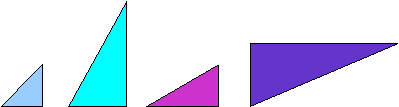Example: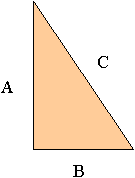For the right triangle above, the lengths of the legs are A and B, and the hypotenuse has length C. Using the Pythagorean Theorem, we know that A2 + B2 = C2.

Example: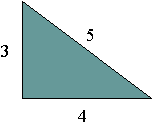In the right triangle above, the hypotenuse has length 5, and we see that 32 + 42 = 52 according to the Pythagorean Theorem.

The database is protected by copyright ©dentisty.org 2016
send message

Main page